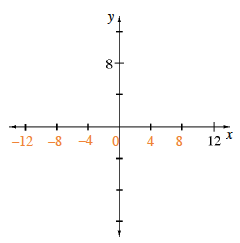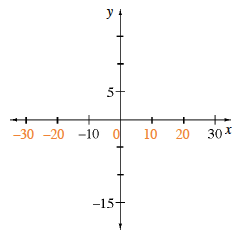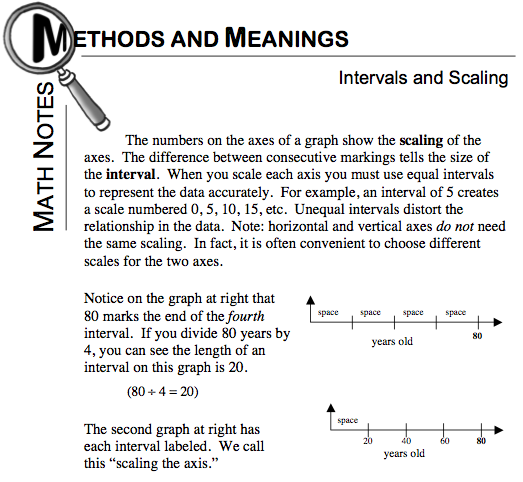Home > CC2 > Chapter 9 > Lesson 9.1.1 > Problem9-13

9-13.
1. Copy the axes below and complete the scales. Homework Help ✎

1.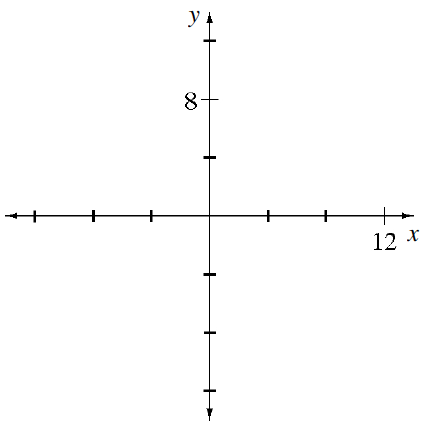2.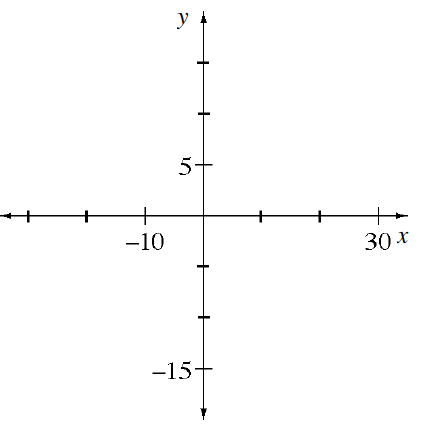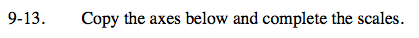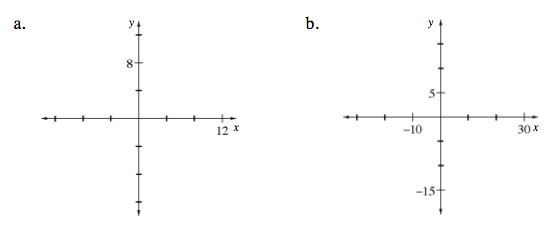For a review of scaling, refer to the Math Notes box below.

Remember that where the x- and y-axes intersect is always 0. To scale the x-axis in part (a), you should try dividing 12 by 3, since that is the number of spaces between 0 and 12.

The x-axes for parts (a) and (b) are labeled below. Were you able to scale the y-axes?